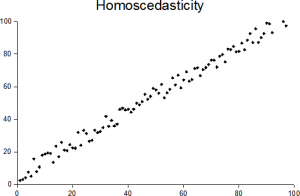# Brown-Forsythe Test: Definition

Share on

## What is the Brown-Forsythe Test?Data with equal variances is evenly dispersed around the line. Image: Sreebot| Wikimedia Commons

The Brown-Forsythe (B-F) Test is for testing the assumption of equal variances in ANOVA. It is a modification of the Levene Test.

## How the Test Works

Both the Levene and B-F tests transform dependent variables for use in an ANOVA test. The only difference between the two tests is in how those transformed variables are constructed. The Levene test uses deviations from group means, which usually results in a highly-skewed set of data; This violates the assumption of normality. The Brown-Forsythe test attempts to correct for this skewness by using deviations from group medians. The result is a test that’s more robust. In other words, the B-F test is less likely than the Levene test to incorrectly declare that the assumption of equal variances has been violated.

The test works as follows:

• The median is calculated for each factor level group.
• The median value is subtracted from each dependent variable in the group.
• An ANOVA is run with the transformed variables. If a factor’s p-value is less than the significance level (usually 5%), the population variances are not equal.

## W or F statistic?

The test statistic used in a regular ANOVA is an F-statistic. The statistic used in an ANOVA with transformed variables is sometimes called a W-Statistic — but it’s really just an F-Statistic with a different name. It should not be confused with the coefficient of concordance W-statistic, which is used to assess agreement between raters.

## Cautions

For the most part, the B-F test is thought to perform as well as or better than other available tests for equal variances. However, Glass and Hopkins (1996 p. 436) state that the Levene and B-F tests are “fatally flawed”; It isn’t clear how robust they are when there is significant differences in variances and unequal sample sizes. Hill et. al (2006) advise repeating the test using a non-parametric method.

References:
Glass, G. & Hopkins, K. (1996). Statistical Methods in Education and Psychology. Needham Heights, MA: Allyn & Bacon.
Hill, T et. al (2006). Statistics: Methods and Applications : a Comprehensive Reference for Science, Industry, and Data Mining. Commercial Statistics.

CITE THIS AS:
Stephanie Glen. "Brown-Forsythe Test: Definition" From StatisticsHowTo.com: Elementary Statistics for the rest of us! https://www.statisticshowto.com/brown-forsythe-test/
---------------------------------------------------------------------------Need help with a homework or test question? With Chegg Study, you can get step-by-step solutions to your questions from an expert in the field. Your first 30 minutes with a Chegg tutor is free!